Function Repository Resource:

# NextSubset

Get the next subset of a given set in canonical order

Contributed by: Wolfram Staff (original content by Sriram V. Pemmaraju and Steven S. Skiena)
 ResourceFunction["NextSubset"][l,s] gives the subset of l following the subset s when the subsets are sorted in canonical order.

## Details and Options

ResourceFunction["NextSubset"] is limited to sets of unique elements.

## Examples

### Basic Examples (1)

Get the subset of {1,2,3} following the subset {1,2} in canonical order:

 In:=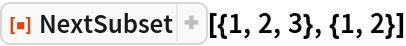Out=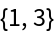### Properties and Relations (2)

Get the subset following another subset in canonical ordering:

 In:=Out=This is not the same as the next subset in lexicographic order, as can be obtained with the resource function NextLexicographicSubset:

 In:=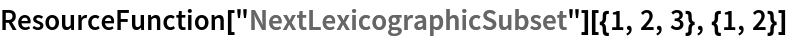Out=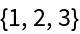The full list of lexicographically-ordered subsets can be obtained using the resource function LexicographicSubsets:

 In:=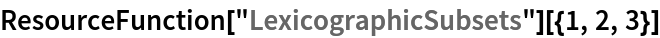Out=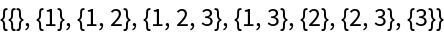Subsets of {1,2,3} in canonical order generated sequentially using NextSubset:

 In:=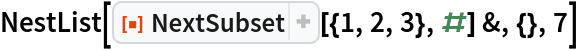Out=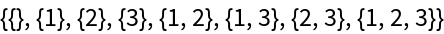Compare:

 In:=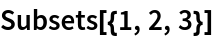Out=In:=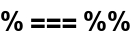Out=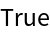## Version History

• 1.0.0 – 15 July 2020